Next: Enciphering matrices Up: fsqFsHn sGGousG Previous: Reading and Writing from

Subsections

# Affine enciphering

We have just examined a trivial encryption scheme, the Caesar cipher, which can be described as applying x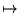x + b (mod p) to each of the characters x in a message. As we have seen, this provides no security at all (a message encoded in this way can be cracked in a short while by a third grader), but minor modifications such as those in §5 can greatly improve the security.

We now return to trivialities, but make it one step harder. Instead of encoding each character by just adding a constant, we can multiply by a constant as well. That is, we can use xax + b (mod p) to encode each character. This not only helps a tiny bit (to crack it, you need to find out two characters instead of just one, and the encoded message looks a little more mixed up'', since letters adjacent in the alphabet no longer encode to adjacent letters), it helps us set up a little more conceptual machinery.

## When do affine encodings fail?

You might be wondering if this will work at all. Certainly when we were just shifting the letters by a fixed amount, there was no chance that the enciphering transformation f could fail to be invertible. Does the same hold true if we multiply the numeric codes for the letters? That is, do different letters always encode to different letters?

The answer is, unfortunately, not always. For example, suppose we use a 27 character alphabet (A-Z and blank). Then if we encode using the transformation x6x + 2 (mod 27), both A''(0) and J''(9) encode to the character C''(2). What went wrong? Recall that when we work modulo 27, any numbers that differ by a multiple of 27 are equivalent. Since 27 has nontrivial factors, several characters will collide whenever the multiplicand (6) shares a common divisor with 27. This will never happen if gcd(a, p) = 1.

Let's make that precise: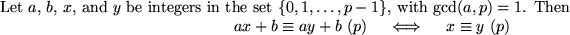Suppose ax + b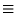ay + b (mod p). We want to show that xy (mod p). Certainly we have axay (mod p), so there must be integers k and m so that kp + ax = mp + ay. But we can then rewrite this as

a(x - y) = (k - m)p

that is, a(x - y) is a multiple of p. If a and p share no common divisors, then x - y must be a multiple of p, so xy (mod p).

If xy (mod p), then certainly ax + bay + b (mod p).

Thus, by the above, we can see that the affine encoding scheme will be just fine if the multiplicand a is relatively prime to the length of our alphabet (p). Henceforth, we will always take an alphabet whose length is a prime number, so we need not worry about this issue. An alphabet of length 97 works quite well, because 97 is prime and large enough so that we can include all the usual printable ASCII characters (see §2.2, especially the table on page). If you plan to use the full ASCII or extended ASCII character set (including the null character), then you will need to make sure you choose a to be an odd number (since the length of the alphabet will be either 128 or 256, both or which are powers of 2).

## Implementing and using an affine encoding

Implementing this scheme is a trivial modification to the Caesar2 procedure we wrote in §4. We only need add another parameter a, and change the encoding calculation. We also renamed shift as b to correspond to our discussion here.

In light of the Prop. 7.1, we will also add some error checking to ensure that a and the length of the alphabet are relatively prime. Recall that if they are not, the encryption will produce a message that cannot be decrypted. We use the error command4.24if this is the case. While we typically use an alphabet of prime length, we may not always do so.

>
AffineCode:= proc(plaintext::string, a::integer, b::integer)
local textnum,codenum,i,p;
global Alphabet;

p       := length(Alphabet);
if (gcd(a,p)>1) then
error("The   2,a,p);
fi;
textnum := StringToList(plaintext);
codenum := [seq( modp(a*textnum[i]+b, p), i=1..length(plaintext)) ];
ListToString(codenum);
end:


>
mess:=AffineCode("A fine mess, Stanley!",10,5);


What about decoding a message encoded by an affine cipher? If we know the original a and b, we could either write AffineDecode, which calculates modp( (textnum[i]-b)/a, p) instead, or, we could use AffineCode(1/a, -b/a), which is really the same thing. We'll take the latter approach.

If you think about it for a second, you may wonder whether 1/a mod p makes any sense at all. It does, but only if we interpret division in the right way. We should not divide by a in the ordinary way'' and then reduce modulo p, but instead interpret it to mean the solution x to the equation ax1 (mod p). That is, 1/a mod p is the multiplicative inverse of a in {0, 1,..., p - 1}. Such an element exists exactly when a and p are relatively prime, as a consequence of Prop. 7.1. Since Maple knows about division modulo p, it allows us to use this notation, which is quite convenient.

>
1/6 mod 97;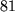In our definition of AffineCode we insisted that a and b be integers. This means that using a call like AffineCode(mess,1/10,-5/10) will give an error, since 1/10 is not an integer. We can fix that by changing the definition of AffineCode to be proc(plaintext::string, a::rational, b::rational) or we can explicitly calculate these modular expressions before passing them to AffineCode.

>
AffineDecode:= proc(ciphertext::string, a::integer, b::integer)
local A, B, p;
global Alphabet;
p := length(Alphabet);
A :=  1/a  mod p;
B := -b/a  mod p;
AffineCode(ciphertext, A, B);
end:


>
AffineDecode(mess,10,5);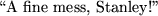## Breaking an affine cipher

Suppose we have a message that was encrypted with an affine cipher, and we also know the Alphabet in use. Suppose also that we know (or manage to guess) the encoding of two letters. How do we then decipher the message? It is quite straightforward: since the message was enciphered with xy = (ax + b) mod p, and we have two examples of x and y, we need only solve a pair of linear equations  mod p. This is quite straightforward to do by hand, but Maple will also do it for us.

For example, suppose we receive the message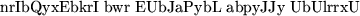which we know is encoded in the 53 letter alphabet consisting of the letters A-Z, a blank, then a-z. Suppose we also guess that the letter b'' (28) is the encoding for a space (26), and that U'' (20) is an encoded s'' (45) . We can figure out the encoding transformation by solving the pair of equations

26a + b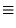28 (mod 53)        45a + b20 (mod 53).

We have Maple do this for us using msolve.
>
msolve(a*26 + b = 28, a*45 +b = 20,53);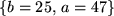Thus, we see that the message was encoded by x47x + 25 (mod 53), and can be decrypted using a = 1/47 (mod 53) and b = - 25/47 (mod 53). Of course, it would have been more efficient to solve for the deciphering transformation directly.

>
msolve(A*28 + B = 26, A*20 +B = 45,53);>
Alphabet:="ABCDEFGHIJKLMNOPQRSTUVWXYZ abcdefghijklmnopqrstuvwxyz":
AffineCode("nrIbQyxEbkrI bwr EUbJaPybL abpyJJy UbUlrrxU", 44, 13);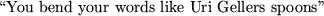#### Footnotes

... command4.24
Versions prior to Maple 6 need to use ERROR instead, which has slightly different behavior.Next: Enciphering matrices Up: fsqFsHn sGGousG Previous: Reading and Writing from

Translated from LaTeX by Scott Sutherland
2002-08-29